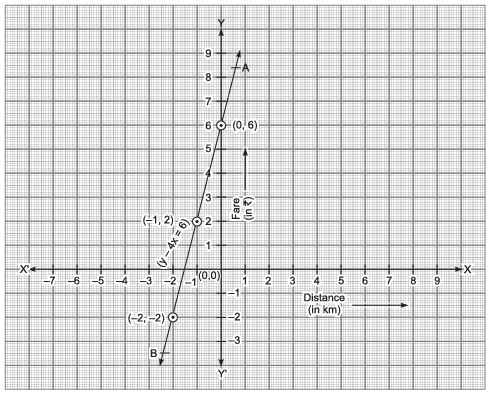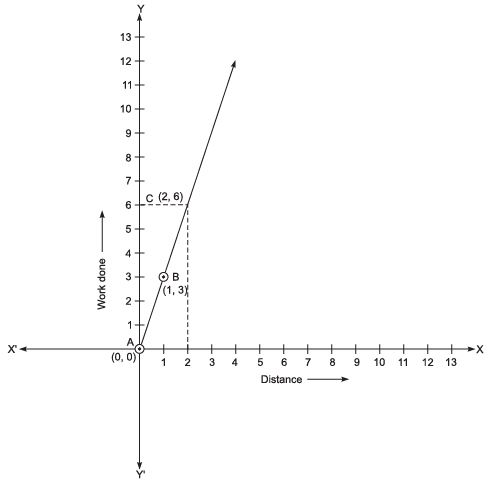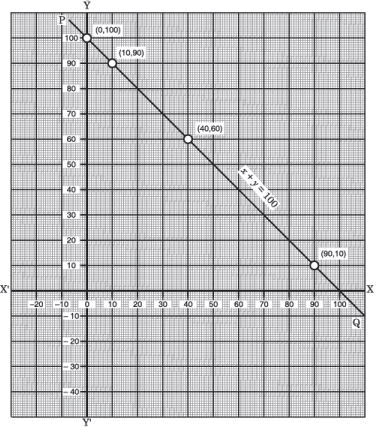# Value Based Questions (VBQs) - Linear Equations in Two Variables Class 9 Notes | EduRev

## Class 9 : Value Based Questions (VBQs) - Linear Equations in Two Variables Class 9 Notes | EduRev

The document Value Based Questions (VBQs) - Linear Equations in Two Variables Class 9 Notes | EduRev is a part of the Class 9 Course Class 9 Mathematics by VP Classes.
All you need of Class 9 at this link: Class 9

Question 1. Kamaruddin is a rikshaw-puller. He charges 10 for the first kilometre and @  4 per kilometre for subsequent distance covered:
(i) Write the linear equation to express the above statement.
(ii) Draw the graph of the linear equation so obtained on a cartesian plane.
(iii) By using a manually operated rikshaw (instead of an autorikshaw), which value is depieted by Kamaruddin?
Solution. (i) Let the total distance covered be x km and the fare charged ₹ y.
∴ Fare for first kilometre = ₹10
Remaining distance = (x – 1) km
⇒ Fare for the remaining distance = ₹ 4 (x – 1)
∴ Total fare = ₹ 10 + ₹ 4 (x – 1)
= ₹ [10 + 4 (x – 1)]
Thus, the required equation is:
y = 10 + 4 (x – 1) = 10 + 4x – 4 = 4x + 6
or
y –4x = 6
(ii) To draw the graph, we use the following table: y – 4x = 6

 x 0 -1 -2 y 6 2 -2 (x,y) (0,6) (-1, 2) (-2,-2)

Plot the points (0, 6), (–1, 2) and (–2, –2). Join the points. The line AB is the required graph.(iii) Promoting pollution free environment.

Question 2. Savita’s car broke down at a point 2 units away from the garage. She requested a man for help, who pushed her car to the garage. The work done by a body on application of a constant force is the product of the constant force and the distance travelled by the body in the direction of force.
(i) Express the above activity in the form of a linear equation in two variables by taking the constant force as 3 units and distance travelled as 2 units.
(ii) Verify it by plotting the graph.
(iii) By pushing the car to the garage, which value is depicted by the man?
Solution. (i) Work done = (constant force) × (distance)
Let workdone be y (units) and distance travelled be x (unit), keeping the force as constant 3 units.
∴  y = 3x which is the required linear equation in variables x and y.

(ii) To plot the graph of linear equation y = 3x,
we need at least two ordered pairs satisfying the equation.
Here, we have : x = 0, y = 0 and x = 1, y = 3.

i.e., ordered pairs (0, 0) and (1, 3) satisfy the equation y = 3x.
Now, we plot the points A (0, 0) and B (1, 3) and join AB as shown below:Note: Here, we have not taken the negative side of the graph, because work done cannot be negative.
To verify the graph, draw a perpendicular to x–axis at (2, 0) meeting the graph AB at the point C.
The co-ordinates of C are (2, 6).
It verifies that the workdone = 6 units.
(iii) Co-operation.

Question 3. A and B, two students of class IX of a school, together contributed 100 towards The Prime Minister’s Relief Fund to help the earthquake victims.
(a) Write a linear equation which the above data satisfies.
(b) Draw the graph for the above data.
(c) Which mathematical concept is used in the above problem?
(d) By donating towards Prime Minister’s Relief Fund, which value is depicted by A and B?
Sol. (a) Let the contribution made by ‘A’ = ₹ x
And the contribution made by ‘B’ = ₹ y
Since, the total contribution made by A and B = ₹100
∴   We have x + y = 100

(b) To draw the graph of x + y = 100 we prepare the following table :

 x 0 10 40 90 y 100 90 60 10 (x,y) (0,100) (10,90) (40,60) (90,10)

Draw x-axis and y-axis, plotting the points (0, 100), (10, 90), (40, 60) and (90, 10) and then joining them, we get a straight line PQ as shown here.
The st. line PQ represents the above data.(c) Linear Equations in two  variables.
(d) Helping the needy persons.

Question 4. The taxi fare in a town is  15 for the first kilometre and 10 per kilometre for the subsequent distance.
(a) Taking the distance as ‘x’ km and total fare as y, write a linear equation for the above information.
(b) Deepak hired a taxi for 10 km and paid  200 for it. He asked the taxi-driver to donate the balance to an orphanage. What amount will the taxi-driver donate to the orphanage?
(c) Which mathematical concept is involved in the above problem?
(d) By asking the taxi-driver to donate the balance to an orphanage, which value is depicted by Deepak?
Sol. (a) Total distance covered = x km
Total taxi fare for ‘x’ km = ₹ y
Taxi fare for first km = ₹ 15
Remaining distance = (x–1) km
Fare for (x – 1) km = ₹ 10 × (x–1)
∴ Total fare = ₹10 (x–1) + 15
⇒ 10 (x – 1) + 15 = y
Which is the required equation representing the above problem                          . ...(1)
(b) Substituting x = 10 in the above equation (1), we get 10 (10 – 1) + 15 = y
⇒ 100 – 1 + 15 = y
⇒ 90 + 15 = y
⇒ y = ₹ 105
As Deepak paid ₹200,
∴ Balance amount = ₹200 – ₹ 105 = ₹ 95
∴ Donation to orphanage = ₹ 95

(c) Linear equations in two variables.

(d) Charity.

Question 5. In a one-day cricket match, the organiser decided to donate as much money to an ‘old -age home’ as the run scored by the first pair of batsman. Ratan and Naval were the opener batsman. They together scored ‘N’ runs such that for every 1 run of Ratan, Naval scored 3 runs.
(a) Set up a linear equation with ‘x’ and ‘y’ representing the number of runs of Ratan and Naval respectively.
(b) Find the number of runs scored by Ratan, if Naval scored 180 runs.
(c) If Ratan’s score is 99, then what amount of money will be donated to the old-age home by the organiser?
(d) Which mathematical concept is used in the above problem?
(e) By making donation to the old-age home, which value is depicted by the organiser?
Sol. (a) Number of runs scored by Ratan = x
∴ Number of runs scored by Naval = y = 3x
[∵ For every 1 run of Ratan, Naval scored 3 runs]
∵ Total number of runs scored together by the opener batsman = N
∴   x + y =N
(b)  ∵ Number of runs scored by Naval = 180
∴ y = 180
Since,
y =3x
⇒ 3x = 180
⇒ x = (180/3) = 60
⇒ Score of Ratan = 60

(c) ∵ Number of runs scored by Ratan, x =99
∴ Number of runs scored by Naval, y =3x = 3 × 99 = 297
Now, x + y =N
∴ N = 99 + 297 = 396
∴ Organisers will donate ₹ 396 to the ‘old age home’.

(d) Linear equations in two variables.
(e) Helping the aged persons.

Offer running on EduRev: Apply code STAYHOME200 to get INR 200 off on our premium plan EduRev Infinity!

132 docs

,

,

,

,

,

,

,

,

,

,

,

,

,

,

,

,

,

,

,

,

,

;